# 网络表示，测度和度量 (Network Representation, Measures & Metrics)

## 复杂网络系列

### 范叶亮 / 2020-11-21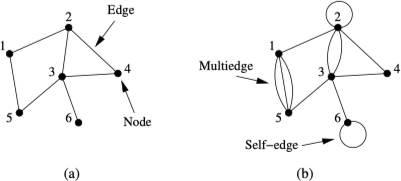## 网络表示

### 无向网络

$$A_{ij}=\left\{\begin{array}{ll} 1 & \text{如果顶点 } i \text{ 和顶点 } j \text{ 之间存在一条边} \\ 0 & \text{其他} \end{array}\right.$$

1. 邻接矩阵对角线上的元素取值均为零。
2. 邻接矩阵是对称的。

### 超图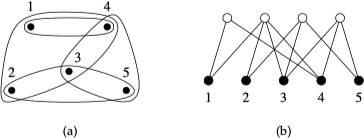### 二分网络

$$B_{ij}=\left\{\begin{array}{ll} 1 & \text{如果顶点 } j \text{ 属于群组 } i \\ 0 & \text{其他} \end{array}\right.$$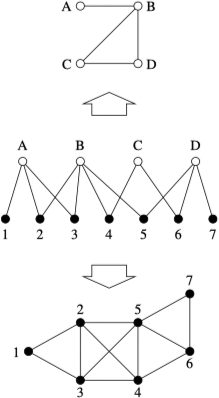### 树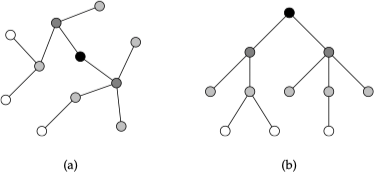### 度

$$k_i = \sum_{j=1}^{n} A_{ij}$$

$$2m = \sum_{j=1}^{n} k_i$$

$$m = \dfrac{1}{2} \sum_{i=1}^{n} k_i = \dfrac{1}{2} \sum_{ij} A_{ij}$$

$$c = \dfrac{1}{n} \sum_{i=1}^{n} k_i$$

$$c = \dfrac{2m}{n}$$

$$\rho = \dfrac{m}{\dbinom{n}{2}} = \dfrac{2m}{n \left(n - 1\right)} = \dfrac{c}{n - 1}$$

$$k_i^{\text{in}} = \sum_{j=1}^{n} A_{ij}, k_j^{\text{out}} = \sum_{i=1}^{n} A_{ij}$$

$$m=\sum_{i=1}^{n} k_{i}^{\mathrm{in}}=\sum_{j=1}^{n} k_{j}^{\mathrm{out}}=\sum_{i j} A_{i j}$$

$$c_{\text {in }}=\frac{1}{n} \sum_{i=1}^{n} k_{i}^{\text {in }}=\frac{1}{n} \sum_{j=1}^{n} k_{j}^{\text {out }}=c_{\text {out }}$$

$$c = \dfrac{m}{n}$$

### 路径

$$N_{ij}^{\left(r\right)} = \left[\mathbf{A}^r\right]_{ij}$$

### 子图

1. 子图（subgraph）：$G'$ 中所有顶点和边均包含于原图 $G$ 中，即 $E' \in E, V' \in V$
2. 生成子图（spanning subgraph）：$G'$ 中顶点同原图 $G$ 相同，且 $E' \in E$
3. 导出子图（induced subgraph）：$G'$ 中，$V' \in V$，同时对于 $V'$ 中任意一个顶点，只要在原图 $G$ 中有对应的边，则也应包含在 $E'$ 中。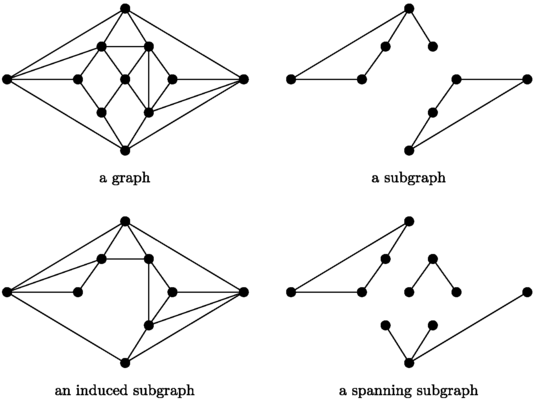### Motif

Motif 2 被定义为反复出现的重要连接模式。这些模式在真实的网络中要比随机网络中出现的更加频繁，如下图所示：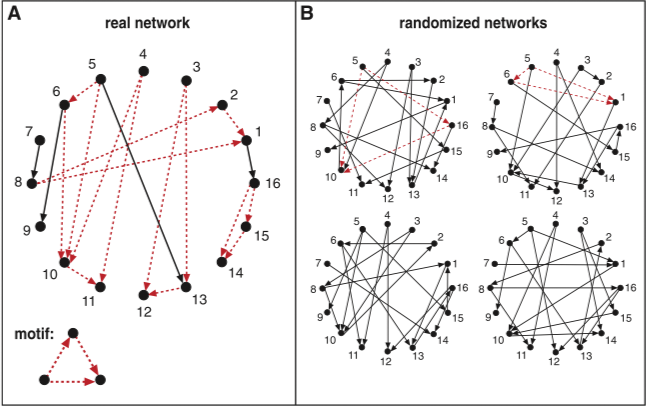Motif 的显著性定义为：

$$Z_i = \dfrac{N_i^{\text{real}} - \bar{N}_i^{\text{rand}}}{\text{std} \left(N_i^{\text{rand}}\right)}$$

### Graphlets

Graphlets 是对 Motif 的扩展，Motif 是从全局的角度发现模式，而 Graphlets 是从局部角度出发。Graphlets 是连接的非同构子图，这里要求子图为导出子图。下图展示了节点数为 2 至 5 的所有 Graphlets：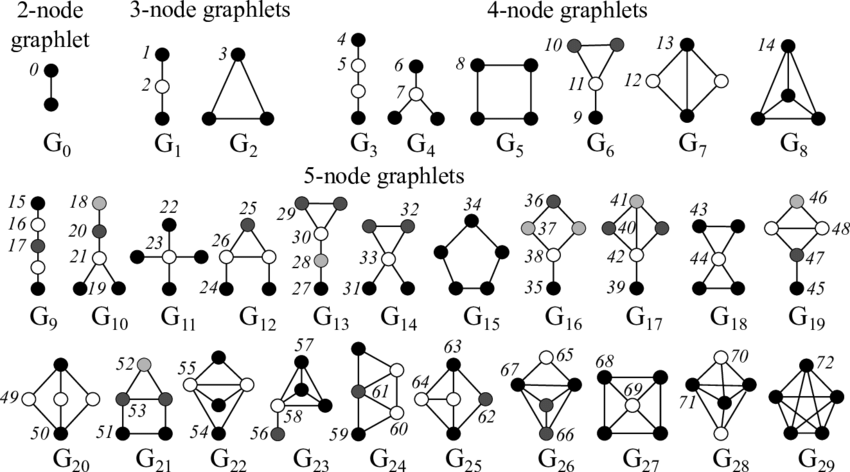## 测度和度量

### 中心性

#### 特征向量中心性

$$x'_i = \sum_{j} A_{ij} x_j$$

$$\mathbf{x} \left(t\right) = \mathbf{A}^t \mathbf{x} \left(0\right)$$

$t \to \infty$ 时，中心性向量的极限与邻接矩阵中的主特征向量成正比。因此，可以等价地认为中心性 $\mathbf{x}$ 满足：

$$\mathbf{A} \mathbf{x} = \kappa_1 \mathbf{x}$$

#### Katz 中心性

Katz 中心性解决了特征向量中心性中节点中心性可能为零的问题。通过为网络中每个顶点赋予少量的“免费”中心性，可以定义：

$$x_i = \alpha \sum_{j} A_{ij} x_j + \beta$$

$$\mathbf{x} = \alpha \mathbf{A} \mathbf{x} + \beta \mathbf{1}$$

$$\mathbf{x} = \left(\mathbf{I} - \alpha \mathbf{A}\right)^{-1} \mathbf{1}$$

#### PageRank

Katz 中心性有一个不足，被一个 Katz 中心性较高的顶点指向的顶点具有较高的 Katz 中心性，但如果这个中心性较高的顶点指向大量顶点，那么这些大量被指向的顶点也会拥有较高的中心性，但这种估计并非总是恰当的。在新的中心性中，那些指向很多其他顶点的顶点，即使本身的中心性很高，但也只能传递给它指向的每个顶点少量的中心性，定义为：

$$x_{i}=\alpha \sum_{j} A_{i j} \frac{x_{j}}{k_{j}^{\text {out }}}+\beta$$

$$\mathbf{x}=\alpha \mathbf{AD}^{-1} \mathbf{x}+\beta \mathbf{1}$$

$$\mathbf{x}=\left(\mathbf{I}-\alpha \mathbf{A} \mathbf{D}^{-1}\right)^{-1} \mathbf{1}$$

PageRank
$\mathbf{x} = \mathbf{A} \mathbf{D}^{-1} \mathbf{x}$

Katz 中心性
$\mathbf{x} = \kappa_1^{-1} \mathbf{A} \mathbf{x}$

#### 接近度中心性

$$C_{i}=\frac{1}{\ell_{i}}=\frac{n}{\sum_{j} d_{i j}}$$

$$C_{i}^{\prime}=\frac{1}{n-1} \sum_{j(\neq i)} \frac{1}{d_{i j}}$$

#### 介数中心性

$$x_{i}=\sum_{s t} \frac{n_{s t}^{i}}{g_{s t}}$$

### 传递性

$$C = \dfrac{\text{长度为 2 的路径中闭合路径数}}{\text{长度为 2 的路径数}}$$

$$C_i = \dfrac{\text{顶点 i 的邻居顶点中直接相连的顶点对数}}{\text{顶点 i 的邻居顶点对总数}}$$

$C_i$ 也称为局部聚类系数（local clustering coefficient），该值代表了 $i$ 的朋友之间互为朋友的平均概率。

### 相似性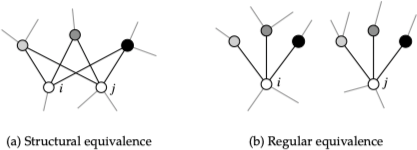#### 结构等价

$$n_{ij} = \sum_{k} A_{ik} A_{kj}$$

$$\sigma_{i j}=\cos \theta=\frac{\sum_{k} A_{i k} A_{k j}}{\sqrt{\sum_{k} A_{i k}^{2}} \sqrt{\Sigma_{k} A_{j k}^{2}}}$$

$$\sigma_{i j}=\frac{\sum_{k} A_{i k} A_{k j}}{\sqrt{k_{i}} \sqrt{k_{j}}}=\frac{n_{i j}}{\sqrt{k_{i} k_{j}}}$$

$$r_{i j}=\frac{\sum_{k}\left(A_{i k}-\left\langle A_{i}\right\rangle\right)\left(A_{j k}-\left\langle A_{j}\right\rangle\right)}{\sqrt{\sum_{k}\left(A_{i k}-\left\langle A_{i}\right\rangle\right)^{2}} \sqrt{\sum_{k}\left(A_{j k}-\left\langle A_{j}\right\rangle\right)^{2}}}$$

#### 规则等价

$$\sigma_{i j}=\alpha \sum_{k l} A_{i k} A_{j l} \sigma_{k l}$$

### 同质性

#### 依据枚举特征的同配混合

$$Q=\frac{1}{2 m} \sum_{i j}\left(A_{i j}-\frac{k_{i} k_{j}}{2 m}\right) \delta_{g_{i} g_{i}}$$

#### 依据标量特征的同配混合

$x_i$ 为顶点 $i$ 的标量值，$\left(x_i, x_j\right)$ 为网络中每一条边 $\left(i, j\right)$ 的两个端点的值，利用协方差可以得到同配系数

$$r=\frac{\sum_{i j}\left(A_{i j}-k_{i} k_{j} / 2 m\right) x_{i} x_{j}}{\sum_{i j}\left(k_{i} \delta_{i j}-k_{i} k_{j} / 2 m\right) x_{i} x_{j}}$$

#### 依据度的同配混合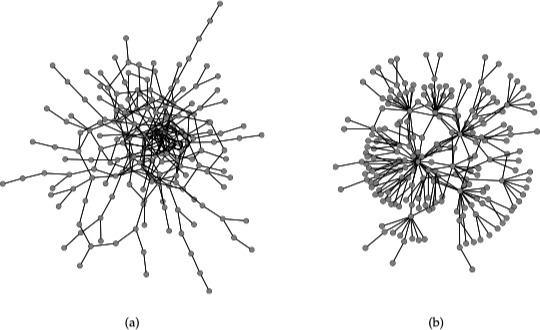1. Newman, M. E. J. (2014) 网络科学引论. 电子工业出版社.
2. Milo, R., Shen-Orr, S., Itzkovitz, S., Kashtan, N., Chklovskii, D., & Alon, U. (2002). Network motifs: simple building blocks of complex networks. Science, 298(5594), 824-827.
3. Jain, D., & Patgiri, R. (2019, April). Network Motifs: A Survey. In International Conference on Advances in Computing and Data Sciences (pp. 80-91). Springer, Singapore.
4. Henderson, K., Gallagher, B., Eliassi-Rad, T., Tong, H., Basu, S., Akoglu, L., … & Li, L. (2012, August). Rolx: structural role extraction & mining in large graphs. In Proceedings of the 18th ACM SIGKDD international conference on Knowledge discovery and data mining (pp. 1231-1239).